# Selina Concise Physics Class 10 ICSE Solutions Electro Magnetism

## Selina Concise Physics Class 10 ICSE Solutions Electro Magnetism

APlusTopper.com provides step by step solutions for Selina Concise ICSE Solutions for Class 10 Physics Chapter 10 Electro-magnetism. You can download the Selina Concise Physics ICSE Solutions for Class 10 with Free PDF download option. Selina Publishers Concise Physics for Class 10 ICSE Solutions all questions are solved and explained by expert teachers as per ICSE board guidelines.

Selina ICSE Solutions for Class 10 Physics Chapter 10 Electro-magnetism

Exercise 10(A)

Solution 1.

Experiment:
In Fig , AB is a wire lying in the north- south direction and connected to a battery through a rheostat and a tapping key. A compass needle is placed just below the wire. It is observed that

1. When the key is open i.e., no current passes through the wire, the needle shows no deflection and it points in the N-S direction (i.e. along the earth’s magnetic field). In this position, the needle is parallel to the wire as shown in Fig. (a).2. When the key is pressed, a current passes in the wire in the direction from A to B (i.e. From south to north) and the north pole(N) of the needle deflects towards the west [Fig. (b)].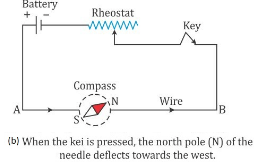3. When the direction of current in the wire is reversed by reversing the connections at the terminals of the battery, North Pole (N) of the needle deflects towards the east [Fig. (c)].4. If the compass needle is placed just above the wire, the North Pole (N) deflects towards east when the direction of current in wire is from A to B [Fig. (d)], but the needle deflects towards west as in fig (e), if the direction of current in wire is from B to A.The above observations of the experiment suggest that a current carrying wire produces a magnetic field around it.

Solution 2.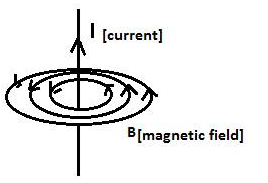Solution 3.

(a) On decreasing the current the magnetic field lines become rarer.
(b) The direction of magnetic field lines will get reversed.

Solution 4.

Right hand thumb rule determines the direction of magnetic field around a current carrying wire.
It states that if we hold the current carrying conductor in right hand such that the thumb points in the direction of flow of current, then the fingers encircle the wire in the direction of the magnetic fields lines.

Solution 5.

(a) The direction of magnetic field at a point just underneath is towards east.
(b) Right hand thumb rule.

Solution 6.

A current carrying conductor produces a magnetic field around it and the magnetic needle in this magnetic field experience a torque due to which it deflects to align itself in the direction of magnetic field.

Solution 7.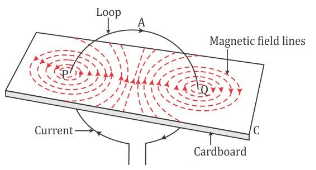Solution 8.

Face of the coil exhibit North polarity.

Solution 9.

(i) Along the axis of coil inwards.
(ii) Along the axis of coil outwards.

Solution 10.Solution 11.

Right hand thumb rule: If we hold the current carrying conductor in right hand such that the thumb points in the direction of flow of current, then the fingers encircle the wire in the direction of the magnetic fields lines.

Solution 12.

(a) A – North pole, B – South pole.
(b) The magnet will be repelled because the end of the solenoid near the north pole of magnet becomes the north pole as current at this face is anticlockwise and the two like poles repel.

Solution 13.

(a) A – North pole, B – South pole.
(b) The north pole of compass needle will deflect towards west.
Reason: The end A of the coil behaves like north pole which repels north pole of compass needle towards west.

Solution 14.

The magnetic field due to a solenoid can be made stronger by using:

1. By increasing the number of turns of winding in the solenoid.
2. By increasing the current through the solenoid.

Solution 15.

A current carrying freely suspended solenoid at rest behaves like a bar magnet. It is because a current carrying solenoid behaves like a bar magnet.

Solution 16.

The needle of the compass will rest in in the direction of magnetic field due to solenoid at that point.

Solution 17.

Magnetic fielddue to a solenoid carrying current increases if a soft iron bar is introduced inside the solenoid.

Solution 18.

(A) When current flows in a wire, it creates magnetic field around it.
(B) On reserving the direction of current in a wire, the magnetic field produced by it gets reversed.
(C) A current carrying solenoid behaves like a bar magnet
(D) A current carrying solenoid when freely suspended, it always rest in north-south direction.

Solution 19.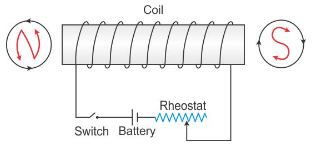Solution 20.

(a) X-north pole, Y –south pole.
(b) By reducing resistance of circuit by mean of rheostat to increase current.

Solution 21.

(a) Solenoid is a cylindrical coil of diameter less than its length.
(b) The device so obtained is electromagnet.
(c) It is used in electric relay.

Solution 22.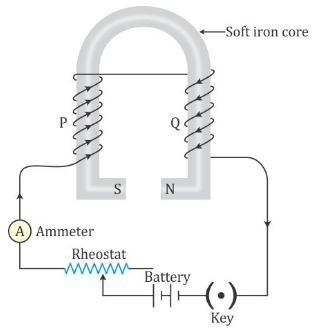Solution 23.

An electromagnet is a temporary strong magnet made from a piece of soft iron when current flows in the coil wound around it. It is an artificial magnet.

The strength of magnetic field of an electromagnet depends on:

1. Number of turns: The strength of magnetic increases on increasing the number of turns of winding in the solenoid.
2. Current: The strength of magnetic field increases on increasing the current through the solenoid.

Solution 24.

At A-south pole and at B-north pole.

Solution 25.

The strength of an electromagnet can be increased by following ways:

1. By increasing the number of turns of winding in the solenoid.
2. By increasing the current through the solenoid.

Solution 27.

1. An electromagnet can produce a strong magnetic field.
2. The strength of the magnetic field of an electromagnet can easily be changed by changing the current in its solenoid.

Solution 28.

 Electromagnet Permanent magnet It is made up of soft iron It is made up of steel. The magnetic field strength can be changed. The magnetic field strength cannot be changed. The electromagnets of very strong field can be made. The permanent magnets are not so strong.

Solution 29.

The soft iron bar acquires the magnetic properties only when an electric current flows through the solenoid and loses the magnetic properties as the current is switched off. That’s why soft iron is used as the core of the electromagnet in an electric bell.

Solution 30.

If an a.c. source is used in place of battery, the core of electromagnet will get magnetized, but the polarity at its ends will change. Since attraction of armature does not depend on the polarity of electromagnet, so the bell will still ring on pressing the switch.

Solution 31.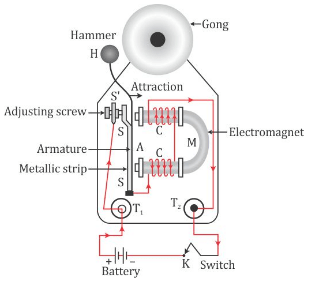Solution 32.

The material used for making the armature of an electric bell is soft iron which can induce magnetism rapidly.

Solution 1 (MCQ).

The presence of magnetic field at a point can be detected by a compass needle.
Note: In the presence of a magnetic field, the needle of compass rests only in the direction of magnetic field and in the absence of any magnetic field, the needle of compass can rest in any direction. In the earth’s magnetic field alone, the needle rests along north-south direction.

Solution 2 (MCQ).

By reversing the direction of current in a wire, the magnetic field produced by it gets reversed in direction.
Hint: On reversing the direction of current in a wire, the polarity of the faces of the wire also reverses. Thus, the direction of magnetic field produced by it also gets reversed.

Exercise 10(B)

Solution 1.

The magnitude of force on a current carrying conductor placed in a magnetic field depends on:

1. On strength of magnetic field B.
2. On current I in the conductor.
3. On length of conductor.

Magnitude of force on a current carrying conductor placed in a magnetic field depends directly on these three factors.

Solution 2.

(a) When current in the conductor is in the direction of magnetic field force will be zero.
(b) When current in the conductor is normal to the magnetic field.

Solution 3.

Direction of force is also reversed.

Solution 4.

Fleming’s left hand rule: Stretch the forefinger, middle finger and the thumb of your left hand mutually perpendicular to each other. If the forefinger indicates the direction of magnetic field and the middle finger indicates the direction of current, then the thumb will indicate the direction of motion of conductor.

Solution 6.

Unit is: Newton/ampere x meter (or NA-1m-1).

Solution 7.

(a) The coil will experience a torque due to which it will rotate.
(b) The coil will come to rest when their plane become normal to the magnetic field.
(c) (i) When plane of a oil is parallel to the magnetic field,
(ii) When plane of coil is normal to the magnetic field.
(d) The instrument which makes use of the principle stated above is d.c. motor.

Solution 8.

(a) The coil begins to rotate in anticlockwise direction.
(b) This is because, after half rotation, the arms AB and CD get interchanged, so the direction of torque on coil reverses. To keep the coil rotating in same direction, commutator is needed to change the direction of current in the coil after each half rotation of coil.Solution 9.

Electric motor: An electric motor is a device which converts the electrical energy into the mechanical energy.
Principle: An electric motor (dc motor) works on the principle that when an electric current is passed through a conductor placed normally in a magnetic field, a force acts on the conductor as a result of which the conductor begins to move and mechanical energy is obtained.

Solution 10.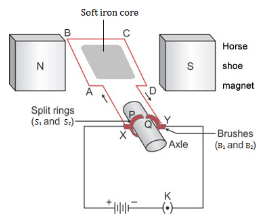Solution 11.

Electrical energy converts into mechanical energy.

Solution 12.

The speed of rotation of an electric motor can be increased by:

1. Increasing the strength of current.
2. Increasing the number of turns in the coil.

Solution 13.

Electric motor is used in electrical gadgets like fan, washing machine, juicer, mixer, grinder etc.

Solution 1 (MCQ).

In an electric motor, the energy transformation is from electrical to mechanical.
Note: An electric motor is a device which converts electrical energy into mechanical energy.

Exercise 10(C)

Solution 1.

(a) Electromagnetic induction: whenever there is change in number of magnetic field lines associated with conductor, an electromotive force is developed between the ends of the conductor which lasts as long as the change is taking place.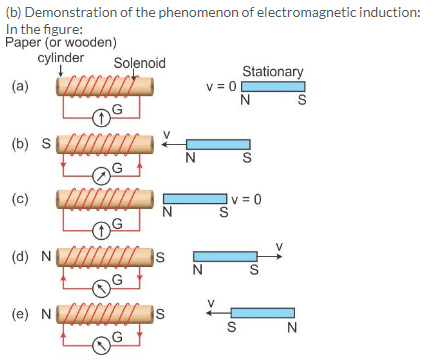1. When the magnet is stationary there is no deflection in galvanometer. The pointer read zero. [Fig. (a)]
2. When the magnetwith north pole facing the solenoid is moved towards the solenoid, the galvanometer shows a deflection towards the right showing that a current flows in the solenoid in the direction as shown in [Fig (b)]
3. As the motion of magnet stops, the pointer of the galvanometer comes to the zero position [Fig (c)]. This shows that the current in the solenoid flows as long as the magnet is moving.
4. If the magnet is moved away from the solenoid, the current again flows in the solenoid, but now in a direction opposite to that shown in [Fig. (b)] and therefore the pointer of the galvanometer deflects towards left[ Fig. (d)].
5. If the magnet is moved away rapidly i.e. with more velocity, the extent of deflection in the galvanometer increases although the direction of deflection remains the same.It shows that more current flows now.
6. If the polarity of the magnet is reversed and then the magnet is brought towards the solenoid, the current in solenoid flows in the direction opposite to that shown in Fig (b) and so the pointer of galvanometer deflect towards left [Fig. (e)].

Solution 2.

Faraday’s formulated two laws of electromagnetic induction:

1. Whenever there is a change in the magnetic flux linked with a coil, an e.m.f. is induced. The induced e.m.f. lasts so long as there is a change in the magnetic flux linked with the coil.
2. The magnitude of the e.m.f. induced is directly proportional to the rate of change of the magnetic flux linked with the coil. If the rate of change of magnetic flux remains uniform, a steady e.m.f. is induced.

Solution 3.

Magnitude of induced e.m.f depend upon:

1. The change in the magnetic flux.
2. The time in which the magnetic flux changes.

Solution 4.

(a) Mechanical energy changes to the electrical energy.
(b) Phenomenon is called electromagnetic induction.

Solution 5.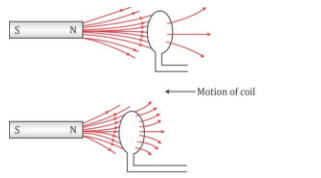(a) When there is a relative motion between the coil and the magnet, the magnetic flux linked with the coil changes. If the north pole of the magnet is moved towards the coil, the magnetic flux through the coil increases as shown in above figure. Due to change in the magnetic flux linked with the coil, an e.m.f. is induced in the coil. This e.m.f. causes a current to flow in the coil if the circuit of the coil is closed.
(b) The source of energy associated with the current obtained in part (a) is mechanical energy.

Solution 6.1. When the magnet is stationary there is no deflection in galvanometer. The pointer read zero. [Fig (a)]
2. When the magnetwith north pole facing the solenoid is moved towards the solenoid, the galvanometer shows a deflection towards the right showing that a current flows in the solenoid in the direction as shown in Fig (b)
3. As the motion of magnet stops, the pointer of the galvanometer to the zero position [Fig (c)]. This shows that the current in the solenoid flows as long as the magnet is moving.
4. If the magnet is moved away from the solenoid , the current again flows in the solenoid , but now in the direction opposite to that shown in [Fig. (b)] and therefore the pointer of the galvanometer deflects towards left [Fig. (d)].
5. If the magnet is moved away rapidly i.e. with more velocity, the extent of deflection in the galvanometer increases although the direction of deflection remains the same.It shows that more current flows now.
6. If the polarity of the magnet is reversed and then the magnet is brought towards the solenoid, the current in solenoid flows in the direction opposite to that shown in Fig (b) and so the pointer of galvanometer deflect towards left [Fig. (e)].

(b) Magnitude of induced e.m.f depend upon:

1. The change in the magnetic flux.
2. The time in which the magnetic flux changes.

(c) The direction of induced e.m.f depends on whether there is an increase or decrease in the magnetic flux.

Solution 7.

The current induced in a closed circuit only if there is change in number of magnetic field lines linked with the circuit.

Solution 8.

1. Yes.
2. Yes.
3. Yes.
4. No.

Solution 9.

Fleming’s right hand rule determines the direction of current induced in the conductor.

Solution 10.

Fleming’s right hand rule: Stretch the forefinger, middle finger and the thumb of your right hand mutually perpendicular to each other. If the forefinger indicates the direction of magnetic field and the thumb will indicates the direction of motion of conductor, then the middle finger indicates the direction of induced current.

Solution 11.

Lenz’s law: It states that the direction of induced e.m.f. (or induced current) is such that it always tends to oppose the cause which produces it.

Solution 12.

When a coil has a large number of turns, then magnitude of induced e.m.f. in the coil become more and then by Lenz’s law it will oppose more.

Solution 13.

So that the mechanical energy spent in producing the change, is transformed into the electrical energy in form of induced current.

Solution 14.

Lenz’s law implies the law of conservation of energy. It shows that the mechanical energy spent in doing work, against the opposing force experienced by the moving magnet, is transformed into the electrical energy due to which current flows in the solenoid.

Solution 15.

The pointer of galvanometer deflects. The deflection last so long as the coil moves.

(a) Deflection becomes twice.
(b) Deflection becomes thrice

Solution 16.

1. The pointer of galvanometer deflects towards left. The deflection lasts so long as the coil moves.
2. (a) Deflection becomes twice (b) Deflection becomes thrice.

Solution 17.

(a)

1. When switch is closed suddenly, the galvanometer needle deflects for a moment.
2. If switch is kept closed then galvanometer needle returns to zero.
3. If switch is opened again then galvanometer needle deflects again but in opposite to the direction of deflection in case (a).

(b) This can be explained by Faraday’s law which states that whenever there is change in the magnetic flux linked with a coil, an e.m.f. is induced. The induced e.m.f. lasts as long as there is a change in the magnetic flux linked with the coil.

Solution 18.

An A.C. generator works on the principle of ‘electromagnetic induction’.
Statement: Whenever a coil is rotated in a magnetic field, the magnetic flux linked with the coil changes, and therefore, an EMF is induced between the ends of the coil. Thus, a generator acts like a source of current if an external circuit containing load is connected between the ends of its coil.

Solution 19.

The number of rotations of the coil in one second or the speed of rotation of the coil.

Solution 20.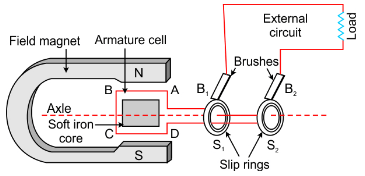Solution 21.

(a)In an a.c generator, if the speed at which the coil rotates is doubled, the frequency is also doubled.
(b) Maximum output voltage is also doubled.

Solution 22.

Two ways in an a.c generator to produce a higher e.m.f. are:

1. By increasing the speed of rotation of the coil.
2. By increasing the number of turns of coil.

Solution 23.

Mechanical energy changes into the electrical energy.

Solution 24.

Two dissimilarities between D.C. motor and A.C. generator:

 A.C. Generator D.C. Motor 1. A generator is a device which converts mechanical energy into electrical energy. 1. A D.C. motor is a device which converts electrical energy into mechanical energy. 2. A generator works on the principle of electromagnetic induction. 2. A D.C. works on the principle of force acting on a current carrying conductor placed in a magnetic field.

Similarity: Both in A.C generator and D.C motor, a coil rotates in a magnetic field between the pole pieces of a powerful electromagnet.

Solution 25.

The voltage of a.c. can be stepped up by the use of step-up transformer at the power generating station before transmitting it over long distances. It reduces the loss of electrical energy as heat in the transmission line wires. On the other hand, if d.c. is generated at the power generating station, its voltage cannot be increased for transmission, and so due to passage of high current in the transmission line wires, there will be a huge loss of electrical energy as heat in the line wires.

Solution 26.

The purpose of the transformer is to step up or step down the a.c. voltage.
No, a transformer cannot be used with a direct current source.

Solution 28.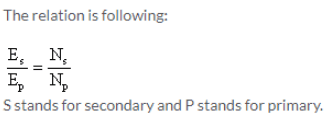Solution 29.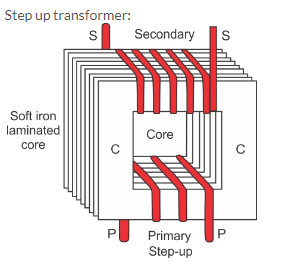Solution 30.

The device is step up transformer.
It works on the principle of electromagnetic induction.

Solution 31.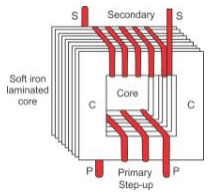Step-up transformer: The step-up transformer is used to change a low voltage alternating e.m.f. to a high voltage alternating e.m.f. of same frequency.

Working: When the terminals of primary coil are connected to the source of alternating e.m.f., a varying current flows through it which also produces a varying magnetic field in the core of the transformer. Thus, the magnetic field lines linked with the secondary coil vary and induce an e.m.f. in the secondary coil. The induced e.m.f. varies in the same manner as the applied e.m.f. in the primary coil varies, and thus, has the same frequency as that of the applied e.m.f.
The magnitude of e.m.f. induced in the secondary coil depends on the ‘turns ratio’ and the magnitude of the applied e.m.f.
For a transformer,Two characteristics of the primary coil as compared to its secondary coil:

1. The number of turns in the primary coil is less than the number of turns in the secondary coil.
2. A thicker wire is used in the primary coil as compared to that in the secondary coil.

Solution 32.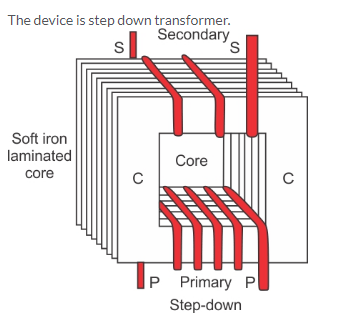Working: In a step down transformer, the number of turns in secondary coil are less than the number of turns in the primary coil i.e., turns ratio NS/NP<1.
As Es/Ep = NS/NP.
So Es/Eps is less than Ep.

Two uses of step down transformer are:

1. With electric bells
2. At the power sub-stations to step-down the voltage before its distribution to the customers.

Solution 34.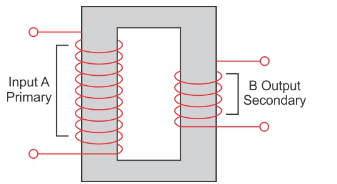A is the primary coil. B is the secondary coil.
We have drawn laminated core in the diagram.
The material of this part is soft iron.
This transformer a step-down transformer because the number of turns in primary coil is much greater than that in the secondary coil.

Solution 35.

(a) Soft iron core is used. The core is made up from the thin laminated sheets of soft iron of T and U shape, placed alternately one above the other and insulated from each other by paint or varnish coating over them.Solution 36.

The secondary windings of a transformer in which the voltage is stepped down are usually made up of thicker than the primary because more current flows in the secondary coil. The use of thicker wire reduces its resistance and therefore the loss of energy as heat in the coil.

Solution 37.

To reduce the energy losses due to eddy currents.

Solution 38.

1. In a step-up transformer, the number of turns in the primary is less than the number of turns in the secondary.
2. The transformer is used in alternating current circuits.
3. In a transformer, the frequency of A.C. voltage remain same.

Solution 39.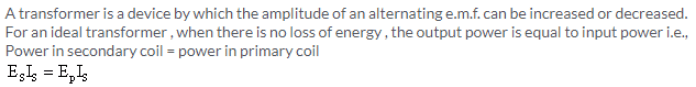Solution 40.

The energy loss in a transformer is called ‘copper loss’.

Copper losses: Primary and secondary coils of a transformer are generally made of copper wire. These copper wires have resistance. When current flows through these wires, a part of the energy is lost in the form of heat. This energy lost through the windings of the transformer is known as copper loss.

This loss can be minimized by using thick wires for the windings. Use of thick wire reduces its resistance and therefore reduces the loss of energy as heat in the coil.

Solution 41.

 Step up transformer Step down transformer It increases the a.c. voltage and decrease the current. It decreases the a.c. voltage and increase the current. The wire of primary coil is thicker than that in the secondary coil. The wire in the secondary coil is thicker than that in the primary coil.

Solution 42.

Soft iron is used in all.

Solution 1 (MCQ).

Fleming’s right hand rule
Statement: According to Fleming’s right hand rule, if we stretch the thumb, middle finger and forefinger of our right hand mutually perpendicular to each other such that the forefinger indicates the direction of magnetic field and thumb indicates the direction of motion of conductor, then the middle finger will indicate the direction of induced current.

Solution 2 (MCQ).

N> NP
Hint: Since a step-up transformer is used to change a low voltage alternating e.m.f. to a high voltage alternating e.m.f. of same frequency, the number of turns in the secondary coil is more than the number of turns in the primary coil, i.e. N> NP.

Numericals

Solution 2.Solution 3.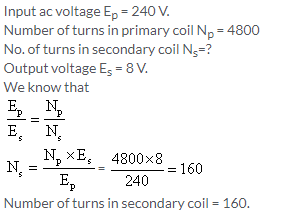Solution 4.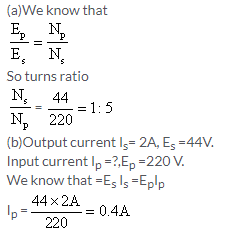More Resources for Selina Concise Class 10 ICSE Solutions